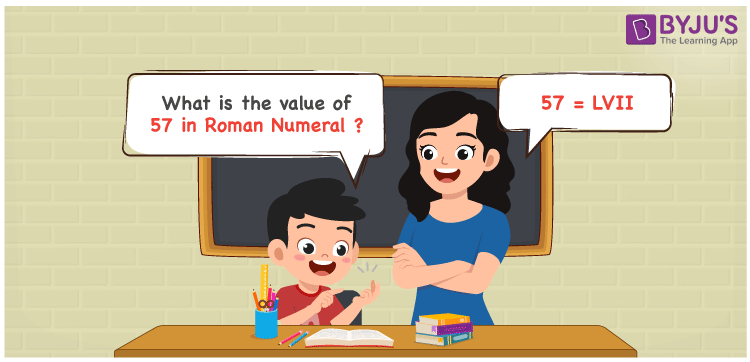# 57 in Roman Numerals

57 in Roman Numerals is LVII. The method of expressing the numbers using the Roman alphabet is known as Roman Numerals. Students can learn how to convert the numbers from 1 to 100 with ease by downloading Roman Numerals 1 to 100 anytime free of cost. In this article, we will learn how to represent the number 57 in Roman Numerals in a simple way.

 Number Roman Numeral 57 LVII

## How to Write 57 in Roman Numerals?We can use the below method to write the number 57 in Roman Numerals

• First, break the number 57 into its least expandable form
• 57 = 50 + 5 + 1 + 1
• Now, write their respective Roman Numerals and add/subtract them
• 57 = L + V + I + I = LVII
• Hence, the value of 57 in Roman Numerals is LVII

## Video Lesson on Roman Numerals## Frequently Asked Questions on 57 in Roman Numerals

Q1

### Write 57 in Roman Numerals.

57 in Roman Numerals is written as LVII.
Q2

### What is the difference between 79 and 57 in Roman Numerals?

79 – 57 = 22. To denote 22 in Roman Numerals, we express 22 in its expanded form i.e., 22 = 10 + 10 + 1 + 1 = X + X + I + I = XXII. Hence the difference between 79 and 57 in Roman Numerals is XXII.
Q3

### What is the value of 50 + 7 in Roman Numerals?

50 + 7 = L + VII = LVII. Therefore the value of 50 + 7 in Roman Numerals is LVII.